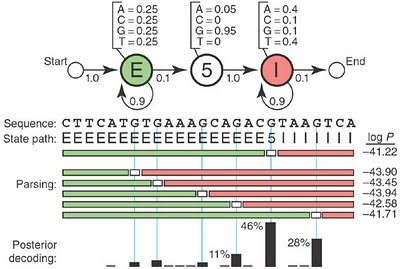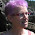## Field of Science

• 6 days ago in The Phytophactor
• 1 month ago in Pleiotropy
• 8 months ago in PLEKTIX
• 8 months ago in History of Geology
• 1 year ago in Angry by Choice
• 2 years ago in Chinleana
• 2 years ago in Field Notes
• 3 years ago in Moss Plants and More
• 4 years ago in Rule of 6ix
• 4 years ago in Games with Words
• 6 years ago in Protein Evolution and Other Musings
• 6 years ago in The View from a Microbiologist
• 6 years ago in Memoirs of a Defective Brain
• 9 years ago in Sex, Genes & Evolution
• 9 years ago in Life of a Lab Rat
• 9 years ago in Disease Prone
• in The Biology Files

### Hidden Markov Models for dummies?

The postdoc just gave me a copy of a short article by Sean Eddy titled "What is a hidden Markov model" (Nature Biotechnology 22: 315-316).  It's only two pages long, and the heading "Primer" flags it as something for beginners.  But I'm struggling to understand it, even with help from the postdoc.  So this post will probably be a mix of attempts to explain what a hidden Markov model (HMM) is and does, and complaints that Eddy has failed to weed out much of the jargon from his explanation.

Below I've pasted the figure he uses to illustrate his explanation.  We assume that we have a short DNA sequence sequence (look in the middle of the figure, below the arrows), and we want to infer which of the bases in it are exon, 5' splice site, or intron.  Because we're told that the sequence starts as exon and contains only one 5' splice site, the only decision we need to make is the location of this splice site.I think this is how an HMM would do this.  It independently considers all of the possible functions for every position, assigns them probabilities (based on the base it finds in the given sequence at that position), and then picks the combination of functions with the best probability score.  Because there are 24 bases and 3 possible functions for each, there are (I think) 3^24 different combinations to be considered.  Fortunately many of these never arise because of several constraints that the model has been given.  First, only Gs and As can be 5' splice sites, as shown in the base-probabilities given at the top of the figure.  Second, there can be only one splice site.  Third the 'exon' function ('E') can only occur before the splice site ('5'), and the 'intron' function ('I') can only occur after it.  This last constraint is indicated by the horizontal and circular arrows that connect these symbols (below the base probabilities); these specify how the state of one position affects the probabilities associated with states at the next position.

After describing the figure Eddy says 'It's useful to imagine a HMM generating a sequence', but I don't think this is what he means.  Or rather, I suppose that he's using the words 'generating' and 'sequence' in some special sense that he hasn't told the reader about.  By 'sequence' he doesn't seem to mean the sequence of bases we were given.  Maybe he means one of the many possible combinations of functions the model will assign to these bases for the purpose of calculating the combination's probability, given the set of constraints the model is using.

He then says 'When we visit a state, we emit a residue from the state's emission probability distribution.'  OK, he did define the 'emission probability distribution' - it's the base probabilities at the top of the figure.  But what can he mean by 'visit a state' and 'emit a residue'?  The postdoc says that 'emit' is jargon that roughly means 'report'.  But we already know the residues - they're the bases of the sequence specified in the figure.  Maybe the HMM is moving along the 24 positions, and at each position it 'visits' it asks what the base is ('emits a residue').  It then considers the probabilities of all three potential states, given both the state assigned to the previous position and the probabilities of finding that specific base given the state it's considering.

Maybe this will make more sense if I consider starting at the 5' end of the sequence and applying the model...

OK, start at position 1.  What states might it have, and with what probabilities?  According to the transition probability arrows, it will have state E with probability 1.0, so we don't need to consider any influence of which base is present at this position (it's a C).  What about the next base (position 2)?  The arrows tell us that there's a 0.1 chance of a transition to state 5, and a 0.9 chance of this position  being in state E like position 2.  The position has base T, which means it can't have state 5 and so must be state E.  The same logic applies to positions 3 and 4 (T and C respectively).

Position 5 has base A, so now we start to consider the first branching of alternatives strings of state assignments, one where position 5 has state E (call this branch A) and one where it has state 5 (call this branch B).  What are the probabilities of these two branches?  To get the probability of the state 5 alternative I guess we multiply the 0.1 probability of a state transition by the 0.05 probability that a state 5 position will have base A.  So the probability of the state 5 branch is only 0.005, which must mean that the probability of the state E branch is 0.995.

Position 6  has base T.  In branch B, this position must have state I, because it follows a state 5 position.  All the bases after this must also be state I, so the total probability of the splice site being at position 5 is 0.005.  In branch A, position 5 must be state E

Position 7 has base G, so we must calculate the probability that it is the splice site as we did for position 5.  We multiply the probability of the transition (0.1) by the probability that a G is the splice site (0.95), giving a branch probability of 0.095 (call this branch C).  But we need to take into account the probability of branch A that we already calculated (0.995), so the total probability of branch C is 0.095 x 0.995 = 0.094525  The other branch can still be called branch A; it has probability 0.995 x 0.905 = 0.900475.  [Quick check - the probabilities so far sum to 1.0 as they should.]

Position 8 is a T; in branch A it must have state E.  Position 9 is another G...   OK, I see where this is going.  I think this example might be a bit too simple because only one branch continues (we don't have to calculate probabilities for multiple simultaneously ramifying branches.  There are only 14 possilbe combinations of states, one for each of the As and Gs in the sequence, because only these are potential splice sites.

Anyway...  Did this exercise help me understand what Eddy is trying to explain?  If what I've written above is correct, then yes, I guess I sort of understand the rest of the article (except for the sentences immediately following the ones I quoted above).  If what I've written is wrong, then, of course, no.

In the next paragraph he explains why this is called a Markov chain (because each state depends only on the preceding state, and why it's 'hidden' (because we don't know the true states).  And the later paragraphs are mostly clearer, except for one place where he lapses back in to the jargon about residues being emitted by states.

He explains that the 'posterior decoding' columns at the bottom of the figure are the probabilities that each of the Gs is the true splice site.  But the probability I've calculated for position 7 (0.095) is larger than indicated by the corresponding column (about 0.03-0.04?), so I might have done something wrong in calculating the probability for this splice site.

Aha.  I've overlooked the different probabilities for the bases in the I state.  I think I have to modify the probability that positions 5 and 7 are splice sites by the probabilities that the bases that follow them are introns.  I shouldn't just calculate the probability that position 5 is a splice site from the position 4-to-5 transition probability and the position 5 emission probability for a splice site (p = .005), and then just assume that the following positions are intron sites.  Instead I need to modify the calculated probability of 0.005 by the probabilities associated with each of the following positions being in the intron state, according to their known base identities.

OK, I take back most of my initially harsh criticism of this article.  There's one big flaw in the middle, where he slips into technical jargon, using what appear to be simple English words ('emit', 'visit') with very specialized technical meanings that cannot be inferred from the context.  But otherwise it's good.

#### 4 comments:

1.Hi Rosie,
HMMs are fun and useful, but I am not sure I like the paper. The system is very, very simple, yet not explained in quite enough detail for me to confirm the numbers (I do get something in the ballpark of e^-41, though).

There are only 24 states in this example (24 places where the 5'SS can be), and only 14 (A or G at the 5'SS site) of them have non-zero probability. The numbers given in the 'posterior decoding' line are the (relative) probability for the whole state path with the 5'SS at a particular position, btw, so your direct calculation won't give you the results shown!

mlg
Gerhard

2.Hi Rosie.

Are you still at UBC? Joel Friedman from the department of Computer Science is teaching about Markov Chains in a very intelligible way: you could send your postdoc to take his course.

Also, he is a very nice guy. If you need more explications I am reasonably sure you can discuss it over lunch.

Jeremy

3.Thanks for the suggestions. I don't have any specific plans to use HMMs; I (and the postdoc) just thought it was something I ought to try to understand, at least superficially.

4.The main problem with that example, from a pedagogical point of view, is that you don't need anything so complicated as a hidden Markov model to analyze it; instead, to compute the probability of a given base being the splice site, you can just multiply together the probability of that base being the splice site (0.95 for a G, etc.), the probability of each base to the left being an exon base, and the probability of each base to the right being an intron base. That gives you the relative probabilities; for the absolute probability, add up all the 24 relative probabilities, and divide each relative probability by that sum to get the absolute probability.

Hidden Markov models might be useful in more complicated situations (say, with rules that tried to decipher the amino acids coded for, within the intron, accounting for the probability of each amino acid). But I doubt it; I think you could just multiply together probabilities there, too, albeit in a slightly more complicated way. In any case, it's always irritating to see extra theoretical machinery used with no good cause.

Markup Key:
- <b>bold</b> = bold
- <i>italic</i> = italic
- <a href="http://www.fieldofscience.com/">FoS</a> = FoS